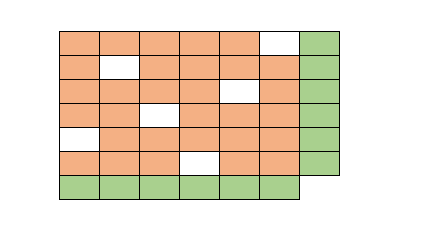## Tuesday, August 29, 2023

### Matrix balancing: introduction

Matrix Balancing Models are often used in economic modeling exercises: they create consistent data sets from data originating from different, conflicting data sources. A standard example is updating a matrix subject to given row and column sums. An example can look like:Update orange cells subject to row/column sums

The empty cells are zero, and they should remain zero. In other words, we need to preserve sparsity. Often, we have non-negativity restrictions on the values. The mathematical model can look like this:

## Friday, August 4, 2023

### Some TSP MTZ experiments

In , a question was posed about a TSP model using the MTZ (Miller-Tucker-Zemlin) subtour elimination constraints. The results with Julia/glpk were disappointing. With $$n=58$$ cities, things were taken so long that the solver seemed to hang. Here I want to see how a precise formulation with a good MIP solver can do better. As seeing is believing, let's do some experiments.

The standard MTZ formulation can be derived easily. We use the binary variables $\color{darkred}x_{i,j}=\begin{cases}1 & \text{if city j is visited directly after going to city i}\\ 0 & \text{otherwise}\end{cases}$